# NCRT Solutions Excercise 4

Question 1: Find the principal and general solutions of the equation tan x=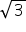Answer 1: tan x=It is known that tanπ/3=and tan (4π/3)= tan(π+ π /3)=tan π/3=Therefore, the principal solutions are x = π/3 and 4π/3.

Now , tan x=tan π/3

⇒ x=nπ+π/3,  where n ∈ Z

Therefore, the general solution is  x=nπ+π/3,  where n ∈ Z

Question 2: Find the principal and general solutions of the equation sec x=2

It is known that sec π/3=2 and sec 5π/3=sec(π2- π/3)=sec π/3=2

Therefore, the principal solutions are x = π/3 and 5π/3 .

Now, sec x = sec π/3

⇒cos x=cos π/3              [sec x =1 cos x]

⇒x=2nπ +π/3, where n ∈ Z

So, the general solution is  x=2nπ +π/3, where n ∈ Z

Question 3: Find the principal and general solutions of the equation    cot x=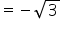Answer 3: cot x=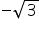As we know cot π/6=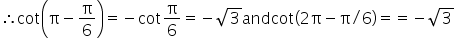i.e.,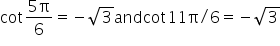Therefore, the principal solutions are x = 5π/6 and 11π/6.

Now, cot x= cot5π/6

⇒tan x =tan5π/6                            [cot x=1/tan x]

⇒x=nπ +5π/6, where n ∈ Z

Thus, the general solution is x=nπ +5π/6, where n ∈ Z

Question 4: Find the general solution of cosec x = –2

Answer 4: cosec x = –2

It is known that

cosec π/6=2

∴cosec (π +π/6)=-2

=>-cosec π/6=-2 and

cosec(2 π -π/6)

=>-cosec π/6=-2

Therefore, the principal solutions are x = 7π/6 and 11π/6 .

Now, cosec x=cosec 7π/6

⇒sin x = sin 7π/6                           [cosec x=1/sin x]

⇒x=nπ +(-1)n  7π/6, where n ∈ Z

Therefore, the general solution is x= nπ +(-1)n  7π/6, where n ∈ Z

Question 5: Find the general solution of the equation cos 4x= cos 2x

Answer 5: cos 4x= cos 2x

⇒cos 4x-cos 2x=0

⇒-2 sin(4x + 2x/2) sin (4x-2x/2)=0

[Formula: cos A-cos B=-2 sin(A+B/2)sin(A-B)]

⇒sin 3x sin x=0

⇒sin 3x=0   or    sin x=0

Therefore   3x=nπ, where   n ∈ Z

⇒ x= nπ /3     or    x= nπ, where   n ∈ Z

Question 6: Find the general solution of the equation cos 3x+cosx-cos 2x=0

⇒2 cos(3x+x/2)cos(3x-x/2)-cos 2x=0      [cos A + cos B=2 cos(A+B/2)cos(A-B/2)]

⇒2 cos 2x cos x-cos 2x=0

⇒cos2x(2 cos x-1)=0

⇒cos 2x = 0             or                    2 cos x-1=0

⇒cos 2x=0               or                    cos x=1/2

Therefore   2x=(2n+1)π/2           or           cos x=cos π/3, where   n ∈ Z

⇒x=(2n+1)π/4                           or                    x=2n±π/3, where   n ∈ Z

Question 7: Find the general solution of the equation sin 2x + cos x=0

Answer 7: sin 2x + cos x=0

⇒ 2 sin x cos x+cos x =0

⇒ cos x (2 sin x +1)=0

⇒ cos x=0               or                    2 sin x + 1=0

Now, cos x=0

⇒cos x=(2n + 1)π/2, where   n ∈ Z

2 sin x + 1=0

⇒sin x=-1/2=-sin π /6=sin(π + π /6)=sin 7π/6

⇒x=nπ+(-1)n 7π/6, where   n ∈ Z

Therefore, the general solution is (2n+1)π/2 or nπ+(-1)n 7π/6, n ∈ Z

Question 8: Find the general solution of the equation sec2 2x=1-tan 2x

⇒1+tan2 2x=1-tan 2x=0

⇒tan2 2x + tan 2x=0

⇒tan 2x (tan 2x +1)=0

⇒tan 2x=0               or                    tan 2x + 1=0

Now, tan 2x=0

⇒tan 2x=tan 0

⇒2x=nπ +0, where   n ∈ Z

⇒x= nπ/2, where   n ∈ Z

tan 2x +1=0

⇒ tan 2x=-1=-tan π/4=tan(π -π/4)=tan 3π/4

⇒2x= nπ + 3π /4, where   n ∈ Z

⇒x= nπ/2 + 3π /4, where   n ∈ Z

Therefore, the general solution is nπ/2 or nπ/2 + 3π/8, n ∈ Z

Question 9: Find the general solution of the equation sin x + sin 3x + sin 5x=0

Answer 9: sin x + sin 3x + sin 5x=0

(sin x + sin 5x) + sin 3x=0

⇒[2 sin(x + 5x/2)cos(x – 5x/2)] + sin 3x=0     [sin A + sin B=2 sin(A+B/2)cos(A-B/2)]

⇒ 2 sin 3x cos(-2x)+sin 3x=0

⇒2 sin 3x cos 2x + sin 3x =0

⇒sin 3x(2 cos 2x + 1)=0

⇒ sin 3x=0   or        2 cos 2x +1=0

Now, sin 3x=0

⇒3x = nπ, where   n ∈ Z

i.e., x= nπ/3, where   n ∈ Z

2 cos 2x + 1=0

⇒ cos 2x=-1/2=-cos π/3=cos(π -π/3)

⇒ cos 2x=cos2π/3

⇒2x=2nπ±2π/3, where   n ∈ Z

⇒x=nπ±2π/3, where   n ∈ Z

Therefore, the general solution is  nπ/3 or nπ±2π/3, n ∈ Z

Related Keywords